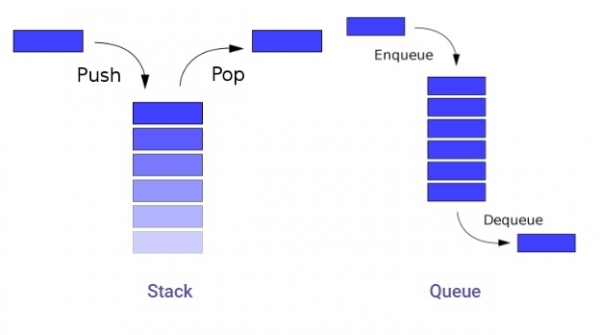# Using List as Stack and Queues in Python

In this article, we will learn about Stack & Queue structures in Python 3.x. Or earlier. Here we will discuss the working and modification within these data structures −

This includes −

1. Insertion operation (Push, Enqueue)
2. Deletion operation (Pop, Dequeue)
3. Display / Traversing Operation

Prerequisites: List & List Operations

Related Data Structure: List Manipulation

## Related Images## Stack

In stacks, objects are stored one over another, and these objects get removed in the reverse order of the arrival i.e. LIFO concept is followed. LIFO means Last in First Out type arrangement is followed in the Stack data structure.

Operations on a Stack −

• Addition / Appending of Element: This increases the stack size by the number of items added and Addition takes place at the upper end i.e. at the top of the stack.
• Deletion / Removal of Element − This involves two conditions − If the Stack is empty no element is available for deletion i.e. Underflow occurs in the Stack or If the Stack has certain elements present in it then the element present at the top gets removed. This reduces the size of the stack by the number of elements removed.
• Traversing /Displaying − This involves visiting each element of the stack and displaying on the screen.

We can also insert an additional functionality of peek i.e. Retrieving the value at the top of the Stack.

## Characteristics of Stack

• Insertion order is preserved.
• Duplicacy is allowed in Stack.
• Similar data-type Storage.
• Highly useful in Parsing Operations.

## Example Code

def isEmpty(stk): # checks whether the stack is empty or not
if stk==[]:
return True
else:
return False

def Push(stk,item): # Allow additions to the stack
stk.append(item)
top=len(stk)-1

def Pop(stk):
if isEmpty(stk): # verifies whether the stack is empty or not
print("Underflow")
else: # Allow deletions from the stack
item=stk.pop()
if len(stk)==0:
top=None
else:
top=len(stk)
print("Popped item is "+str(item))

def Display(stk):
if isEmpty(stk):
print("Stack is empty")
else:
top=len(stk)-1
print("Elements in the stack are: ")
for i in range(top,-1,-1):
print (str(stk[i]))

# executable code
if __name__ == "__main__":
stk=[]
top=None
Push(stk,1)
Push(stk,2)
Push(stk,3)
Push(stk,4)
Pop(stk)
Display(stk)

The above code implements the Stack functionality in Python 3.x. or earlier. We can make a menu-driven program by providing choices to the user by using multiple if-else statements. The concept of framing the Stack remains the same in both cases.

The screen shown below depicts the output produced by the above program. We can also use input() function for user-based input system(Here I implemented static inputs )

## Output

Popped item is 4
Elements in the stack are:
3
2
1

## Queue

In stacks, objects are stored one after another, and these objects get removed in the order of the arrival i.e. FIFO concept is followed. FIFO means First in First Out type arrangement is followed in Queue data structure.

Operations on a Queue

• Addition / Appending of Element − This increases the queue size by the number of items added and Addition takes place at the rear end i.e. at the back of the queue.

• Deletion / Removal of Element − This involves two conditions − If the Queue is empty no element is available for deletion i.e. Underflow occurs in the Queue or If the Queue has certain elements present in it then the element present at the front gets removed. This reduces the size of the stack by the number of elements removed.

• Traversing /Displaying − This involves visiting each element of the stack and displaying on the screen.

We can also insert an additional functionality of peek i.e. Retrieving the value at the back/end of the Queue.

## Characteristics of Queue

• Insertion order is preserved.
• Duplicacy is allowed in Queue.
• Similar data-type Storage.
• Highly useful in Parsing CPU task operations.

## Example Code

Live Demo

#Adding elements to queue at the rear end
def enqueue(data):
queue.insert(0,data)

#Removing the front element from the queue
def dequeue():
if len(queue)>0:
return queue.pop()
return ("Queue Empty!")

#To display the elements of the queue
def display():
print("Elements on queue are:");
for i in range(len(queue)):
print(queue[i])

# executable code
if __name__=="__main__":
queue=[]
enqueue(5)
enqueue(6)
enqueue(9)
enqueue(5)
enqueue(3)
print("Popped Element is: "+str(dequeue()))
display()

The above code implements the Queue functionality in Python 3.x. or earlier. We can make a menu-driven program by providing choices to the user by using multiple if-else statements. The concept of framing the Queue remains the same in both cases.

The screen shown below depicts the output produced by the above program. We can also use input() function for user-based input system(Here I implemented static inputs )

## Output

Popped item is: 5
Elements on queue are:
3
5
9
6

## Conclusion

In this article, we learnt how to implement the Stack & Queue data structure in Python 3.x. Or earlier. You can implement the same algorithm to implement a stack/queue detector program in any other programming language.